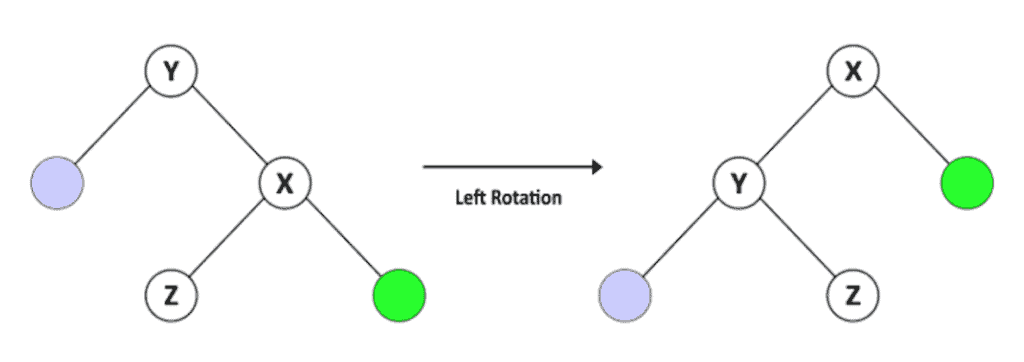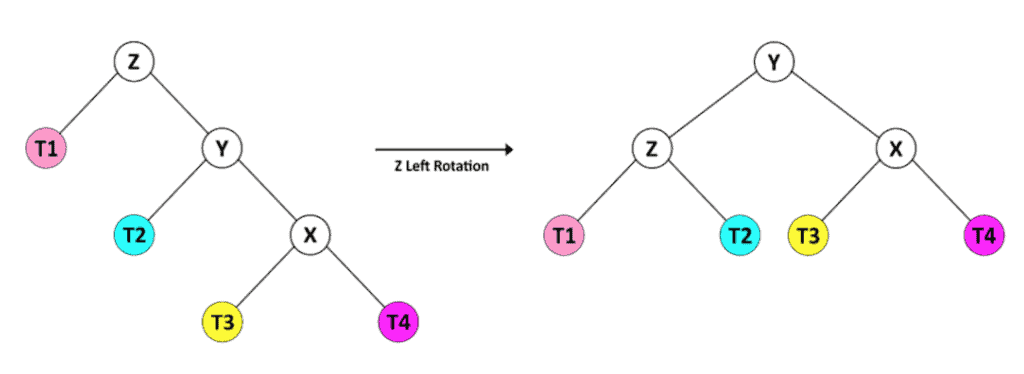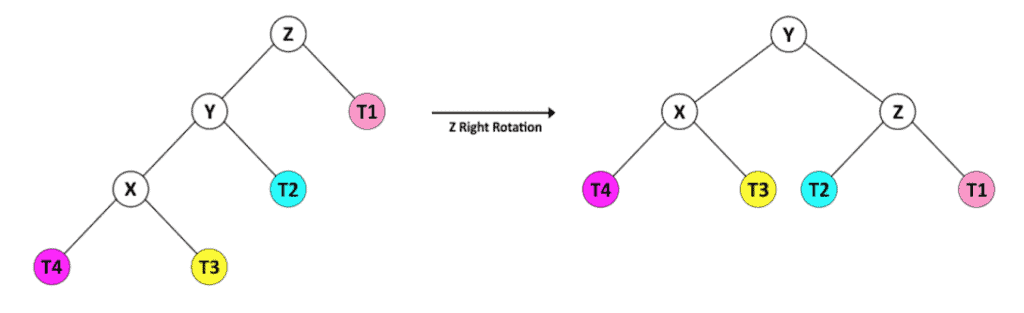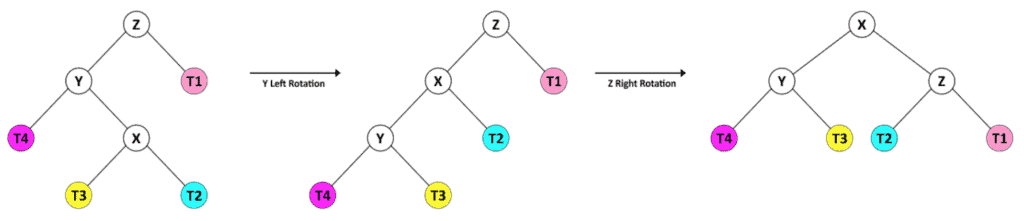### I just announced the new Learn Spring course, focused on the fundamentals of Spring 5 and Spring Boot 2:

>> CHECK OUT THE COURSE

## 1. Introduction

In this tutorial, we'll introduce the AVL Tree and we'll look at algorithms for inserting, deleting, and searching for values.

## 2. What Is AVL Tree?

The AVL Tree, named after its inventors Adelson-Velsky and Landis, is a self-balancing binary search tree (BST).

A self-balancing tree is a binary search tree that balances the height after insertion and deletion according to some balancing rules.

The worst-case time complexity of a BST is a function of the height of the tree. Specifically, the longest path from the root of the tree to a node. For a BST with N nodes, let's say that that every node has only zero or one child. Therefore its height equals N, and the search time in the worst case is O(N). So our main goal in a BST is to keep the maximum height close to log(N).

The balance factor of node N is height(right(N)) – height(left(N)). In an AVL Tree, the balance factor of a node could be only one of 1, 0, or -1 values.

Let's define a Node object for our tree:

``````public class Node {
int key;
int height;
Node left;
Node right;
...
}``````

Next, let's define the AVLTree:

``````public class AVLTree {

private Node root;

void updateHeight(Node n) {
n.height = 1 + Math.max(height(n.left), height(n.right));
}

int height(Node n) {
return n == null ? -1 : n.height;
}

int getBalance(Node n) {
return (n == null) ? 0 : height(n.right) - height(n.left);
}

...
}``````

## 3. How to Balance an AVL Tree?

The AVL Tree checks the balance factor of its nodes after the insertion or deletion of a node. If the balance factor of a node is greater than one or less than -1, the tree rebalances itself.

There are two operations to rebalance a tree:

• right rotation and
• left rotation.

### 3.1. Right Rotation

Assume we have a BST called T1, with Y as the root node, X as the left child of Y, and Z as the right child of X. Given the characteristics of a BST, we know that X < Z < Y.

After a right rotation of Y, we have a tree called T2 with X as the root and Y as the right child of X and Z as the left child of Y. T2 is still a BST because it keeps the order X < Z < Y.Let's take a look at the right rotation operation for our AVLTree:

``````Node rotateRight(Node y) {
Node x = y.left;
Node z = x.right;
x.right = y;
y.left = z;
updateHeight(y);
updateHeight(x);
return x;
}``````

### 3.2. Left Rotation Operation

We also have a left rotation operation.

Assume a BST called T1, with Y as the root node, X as the right child of Y, and Z as the left child of X. Given this, we know that Y < Z < X.

After a left rotation of Y, we have a tree called T2 with X as the root and Y as the left child of X and Z as the right child of Y. T2 is still a BST because it keeps the order Y < Z < X.Let's take a look at the left rotation operation for our AVLTree:

``````Node rotateLeft(Node y) {
Node x = y.right;
Node z = x.left;
x.left = y;
y.right = z;
updateHeight(y);
updateHeight(x);
return x;
}``````

### 3.3. Rebalancing Techniques

We can use the right rotation and left rotation operations in more complex combinations to keep the AVL Tree balanced after any change in its nodes. In an unbalanced structure, at least one node has a balance factor equal to 2 or -2. Let's see how we can balance the tree in these situations.

When the balance factor of node Z is 2, the subtree with Z as the root is in one of these two states, considering Y as the right child of Z.

For the first case, the height in the right child of Y (X) is greater than the hight of the left child (T2). We can rebalance the tree easily by a left rotation of Z.For the second case, the height of the right child of Y (T4) is less than the height of the left child (X). This situation needs a combination of rotation operations.In this case, we first rotate Y to the right, so the tree gets in the same shape as the previous case. Then we can rebalance the tree by a left rotation of Z.

Also, when the balance factor of node Z is -2, its subtree is in one of these two states, so we consider Z as the root and Y as its left child.

The height in the left child of Y is greater than that of its right child, so we balance the tree with the right rotation of Z.Or in the second case, the right child of Y has a greater height than its left child.So, first of all, we transform it into the former shape with a left rotation of Y, then we balance the tree with the right rotation of Z.

Let's take a look at the rebalance operation for our AVLTree:

``````Node rebalance(Node z) {
updateHeight(z);
int balance = getBalance(z);
if (balance > 1) {
if (height(z.right.right) > height(z.right.left)) {
z = rotateLeft(z);
} else {
z.right = rotateRight(z.right);
z = rotateLeft(z);
}
} else if (balance < -1) {
if (height(z.left.left) > height(z.left.right))
z = rotateRight(z);
else {
z.left = rotateLeft(z.left);
z = rotateRight(z);
}
}
return z;
}``````

We'll use rebalance after inserting or deleting a node for all the nodes in the path from the changed node to the root.

## 4. Insert a Node

When we're going to insert a key in the tree, we have to locate its proper position to pass the BST rules. So we start from the root and compare its value with the new key. If the key is greater, we continue to the right — otherwise, we go to the left child.

Once we find the proper parent node, then we add the new key as a node to left or right, depending on the value.

After inserting the node, we have a BST, but it may not be an AVL Tree. Therefore, we check the balance factors and rebalance the BST for all the nodes in the path from the new node to the root.

Let's take a look at the insert operation:

``````Node insert(Node node, int key) {
if (node == null) {
return new Node(key);
} else if (node.key > key) {
node.left = insert(node.left, key);
} else if (node.key < key) {
node.right = insert(node.right, key);
} else {
throw new RuntimeException("duplicate Key!");
}
return rebalance(node);
}``````

It is important to remember that a key is unique in the tree — no two nodes share the same key.

The time complexity of the insert algorithm is a function of the height. Since our tree is balanced, we can assume that time complexity in the worst case is O(log(N)).

## 5. Delete a Node

To delete a key from the tree, we first have to find it in the BST.

After we find the node (called Z), we have to introduce the new candidate to be its replacement in the tree. If Z is a leaf, the candidate is empty. If Z has only one child, this child is the candidate, but if Z has two children, the process is a bit more complicated.

Assume the right child of Z called Y. First, we find the leftmost node of the Y and call it X. Then, we set the new value of Z equal to X ‘s value and continue to delete X from Y.

Finally, we call the rebalance method at the end to keep the BST an AVL Tree.

Here is our delete method:

``````Node delete(Node node, int key) {
if (node == null) {
return node;
} else if (node.key > key) {
node.left = delete(node.left, key);
} else if (node.key < key) {
node.right = delete(node.right, key);
} else {
if (node.left == null || node.right == null) {
node = (node.left == null) ? node.right : node.left;
} else {
Node mostLeftChild = mostLeftChild(node.right);
node.key = mostLeftChild.key;
node.right = delete(node.right, node.key);
}
}
if (node != null) {
node = rebalance(node);
}
return node;
}``````

The time complexity of the delete algorithm is a function of the height of the tree. Similar to the insert method, we can assume that time complexity in the worst case is O(log(N)).

## 6. Search for a Node

Searching for a node in an AVL Tree is the same as with any BST.

Start from the root of the tree and compare the key with the value of the node. If the key equals the value, return the node. If the key is greater, search from the right child, otherwise continue the search from the left child.

The time complexity of the search is a function of the height. We can assume that time complexity in the worst case is O(log(N)).

Let's see the sample code:

``````Node find(int key) {
Node current = root;
while (current != null) {
if (current.key == key) {
break;
}
current = current.key < key ? current.right : current.left;
}
return current;
}``````

## 7. Conclusion

In this tutorial, we have implemented an AVL Tree with insert, delete, and search operations.

As always, you can find the code over on Github.

### I just announced the new Learn Spring course, focused on the fundamentals of Spring 5 and Spring Boot 2:

>> CHECK OUT THE COURSE
Inline Feedbacks